## Nonlinear Least Squares Fitting

Given a functionof a variabletabulated atvalues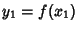, ...,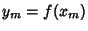, assume the function is of known analytic form depending onparameters, and consider the overdetermined set ofequations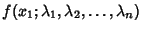(1)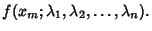(2)

We desire to solve these equations to obtain the values, ...,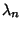which best satisfy this system of equations. Pick an initial guess for theand then define(3)

Now obtain a linearized estimate for the changesneeded to reduce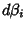to 0,(4)

for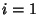, ...,. This can be written in component form as(5)

where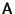is theMatrix(6)

In more concise Matrix form,(7)

whereandare-Vectors. Applying the Matrix Transpose ofto both sides gives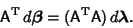(8)

Defining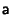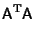(9)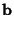(10)

in terms of the known quantitiesandthen gives the Matrix Equation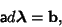(11)

which can be solved forusing standard matrix techniques such as Gaussian Elimination. This offset is then applied toand a new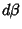is calculated. By iteratively applying this procedure until the elements ofbecome smaller than some prescribed limit, a solution is obtained. Note that the procedure may not converge very well for some functions and also that convergence is often greatly improved by picking initial values close to the best-fit value. The sum of square residuals is given byafter the final iteration.An example of a nonlinear least squares fit to a noisy Gaussian Function(12)

is shown above, where the thin solid curve is the initial guess, the dotted curves are intermediate iterations, and the heavy solid curve is the fit to which the solution converges. The actual parameters are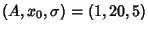, the initial guess was (0.8, 15, 4), and the converged values are (1.03105, 20.1369, 4.86022), with. The Partial Derivatives used to construct the matrixare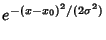(13)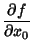(14)(15)

The technique could obviously be generalized to multiple Gaussians, to include slopes, etc., although the convergence properties generally worsen as the number of free parameters is increased.

An analogous technique can be used to solve an overdetermined set of equations. This problem might, for example, arise when solving for the best-fit Euler Angles corresponding to a noisy Rotation Matrix, in which case there are three unknown angles, but nine correlated matrix elements. In such a case, write thedifferent functions as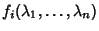for, ...,, call their actual values, and define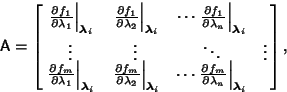(16)

and(17)

whereare the numerical values obtained after theth iteration. Again, set up the equations as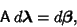(18)

and proceed exactly as before.